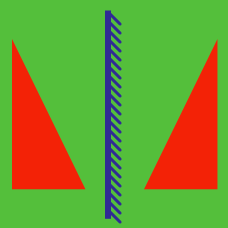Geometry

# Isometries: Level 3 Challenges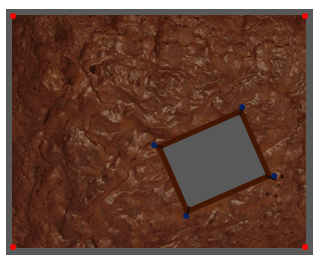I came back from letting my brownies cool to find that some crazy individual had cut themselves a huge rectangular piece out of the middle of my brownie. The piece does not even align with the tray. Is it still possible to use a single straight cut, that is perpendicular to this surface of the brownie, to cut the remaining brownie area into two pieces of equal area?

If not, prove why not. If it's possible, how can you do it?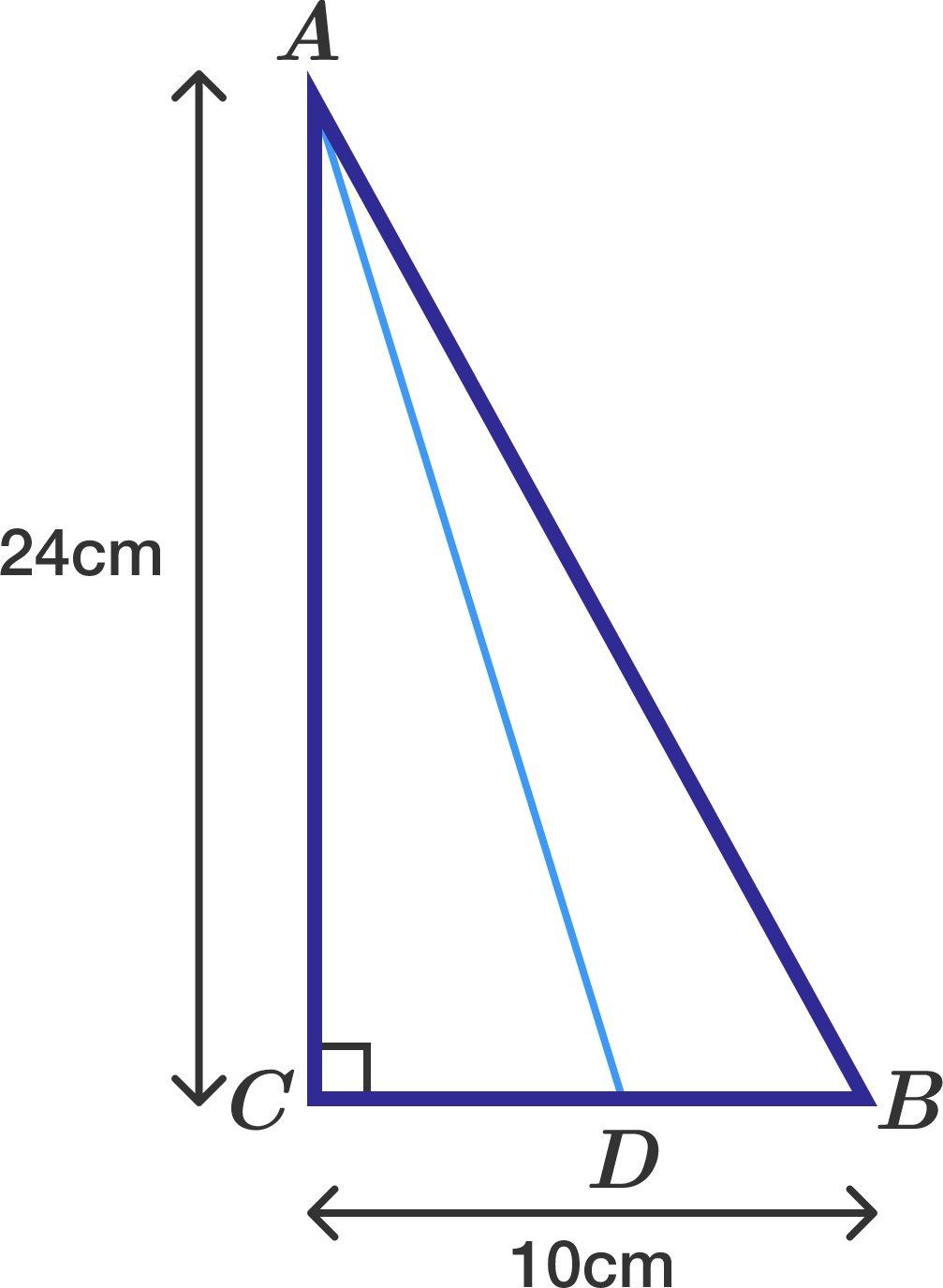A right angle triangle $ABC$ is such that $AC=24$cm, $CB=10$cm and $\angle ACB=90^{\circ}$. A point $D$ on $CB$ is such that when point $C$ is reflected upon line $AD$, the reflected point will be on line $AB$. The length of $CD=\frac{a}{b}$ where $a$ and $b$ are coprime integers. Find the value of $a+b$.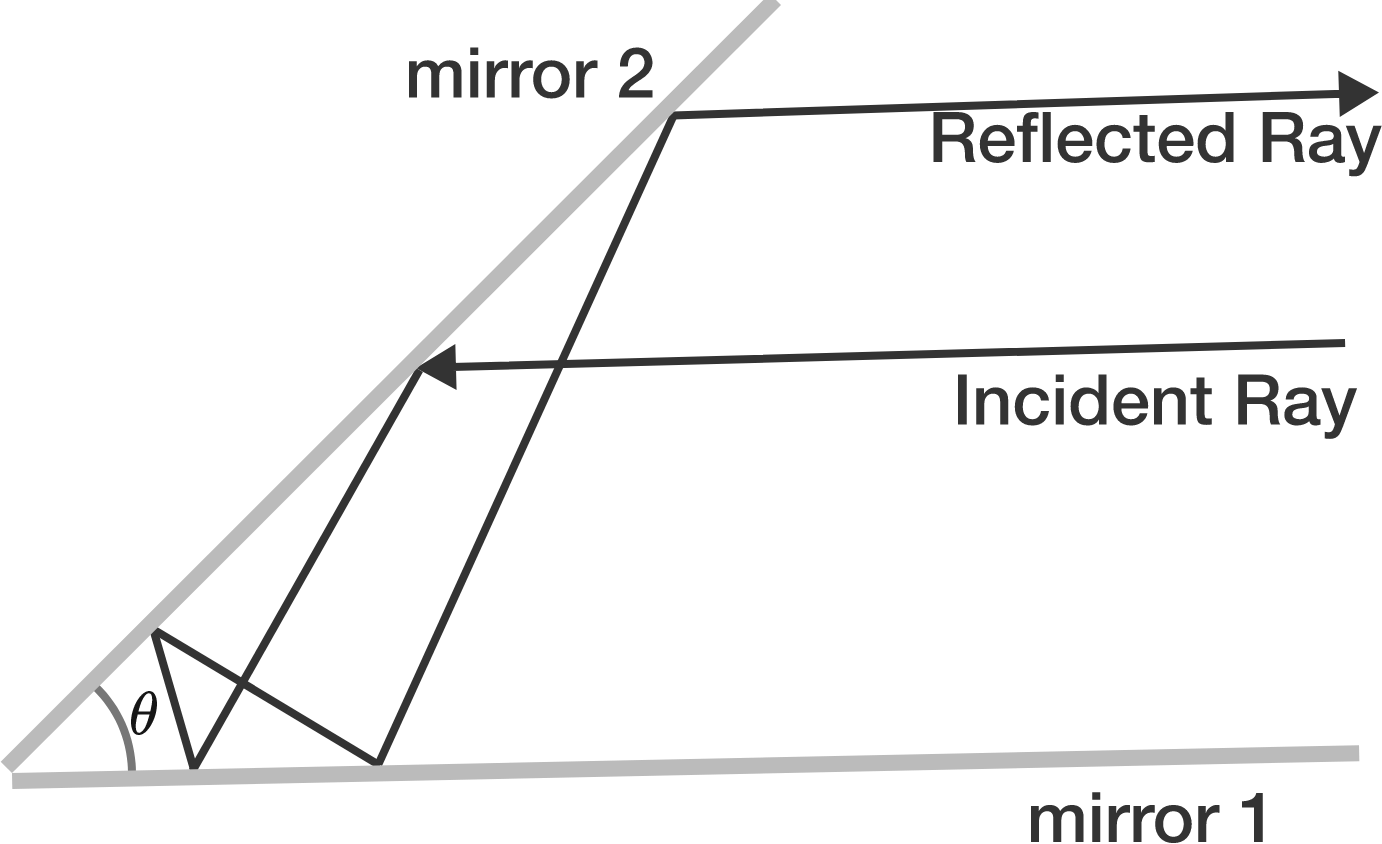The angle between two mirrors is $\theta$. If the incident ray that is parallel to mirror 1 is reflected 5 times in the setup, and becomes parallel to mirror 1 again. What is the measure (in degrees) of $\theta$?

Consider a parabolic mirror with vertex $O$. A light ray originating from focus $S$ of the parabola, strikes the mirror at a point $P$ such that it gets deviated by $30^{\circ}$ after reflection.

Now, if we draw a tangent at point $P$ to the same parabola, it intersects the tangent at vertex at point $Q$. Find $\angle OSQ$ (in degrees).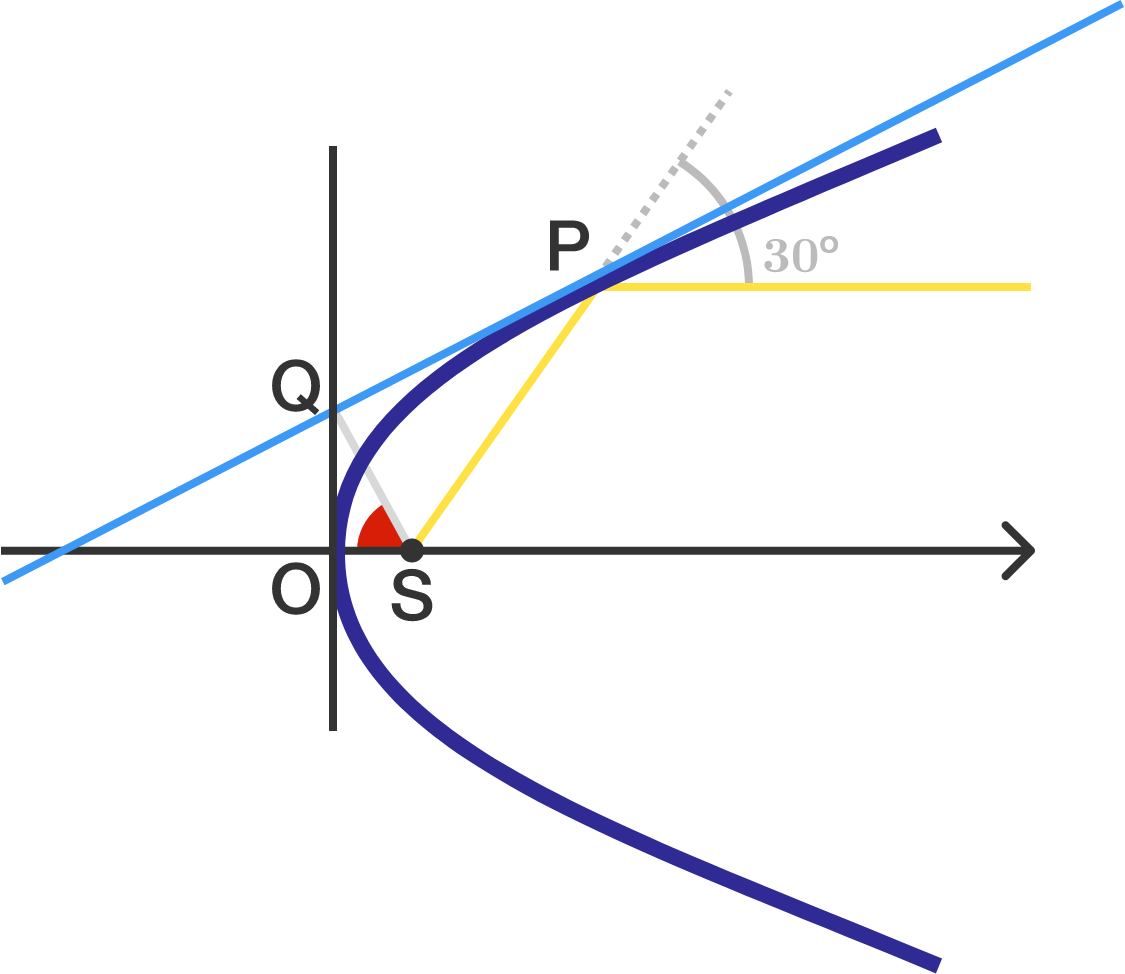The image is not drawn to scale.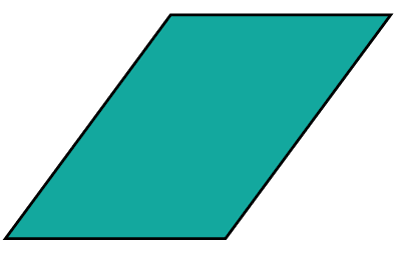How many lines of symmetry does a parallelogram possess?

Note: The parallelogram has no special properties as of a rectangle or a rhombus.

×